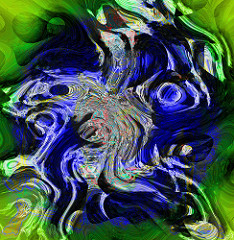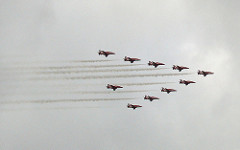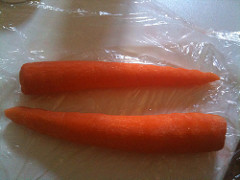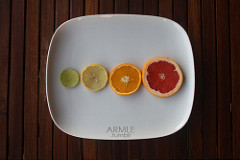# Ch. 4 Organic Chemistry (Study of Chemical Reactions)Marie Florence
question

Thermodynamics

Branch of chemistry concerned with energy changes accompanying chemical and physical transformations. The basic energetics of the reaction at equilibrium.
question

Mechanisms

The step by step description of exactly which bonds break and which bonds form in what order to give the observed products.
question

Kinetics

The study of reaction rates, determine which products are formed the fastest/slowest. Also predicts how rate changes if we change reaction conditions.
question

Quantum yieldThe percent of energy given back as light emission from an excited fluorochrome. Also the ratio of Carbon atoms fixed or Oxygen molecules produced to the number of photons absorbed.
question

Chain Reaction

The mechanism consisting of three steps (initiation, propagation, termination) that explains the formation of a product.
question

Initiation Step

The preliminary step in the chain reaction that generates a reactive intermediate. In chlorination this step leads to the formation of free radicals after chlorine absorbs blue light.
question

Propagation Step

Secondary step in the chain reaction where the reactive intermediate reacts with a stable molecule until the supply of reactants is gone or the reactive intermediate is destroyed. In chlorination it involves the removal of a hydrogen from methane, and the reaction of the methyl radical with Cl⁻ to form CH₃Cl.
question

Termination Step

The third and final step of the chain reaction in which side reactions destroy reactive intermediates(usually free radicals) and tend to stop or slow product formation.
question

Species with unpaired electrons(Cl⁻, Br⁻, OH⁻, CH₃-, CH₃CH₂- for example), that are electron deficient because they lack an octet. These readily combine with electrons in other atoms to form a bond. Are sp² hybridized and planar.
question

Reactive intermediate

A short-lived species that is never present in high concentrations because it reacts as quickly as it is formed.
question

Equilibrium constant

Ratio of the concentrations of the products to the concentrations of the reactants at the point of equilibrium, where each reactant and product in the expression is raised to the power of its stoichiometric coefficient. Signified Keq= e⁻(ΔG/RT)
question

Gibbs Free Energy

The measure of the amount of energy available to do work. Denoted by G, an negative value of delta G means that reactions can occur spontaneously.
question

standard Gibbs free energy

This is the equation for _______ _______ ______ _________ .ΔG⁰ = ∑vΔG⁰(products) – ∑vΔG⁰(reactants), can also equal -RT(ln Keq) or -2.303RT(log Keq)
question

Enthalpy

The change in energy of a chemical reaction Denoted by (ΔH). This is a measure of the relative strength of bonding in the products and reactants.
question

Entropy

A quantitative measure of disorder or randomness, symbolized by S.
question

Chlorination

The substitution reaction of methane(CH₄) with chlorine requires light and heat to take place at a useful rate. The most effective wavelength for this reaction to happen is blue. Very high quantum yield. Forms chloromethane(CH₃Cl) in first step.
question

Einstein

Defined as a mole of photons.
question

Regeneration

The _______________ of a free radical is characteristic of a propagation step in a chain reaction.
question

Bleomycin

An anti-tumor antibiotic which is used for the treatment of testicular cancer. Works by generating highly reactive hydroxyl radicals, which damage and degrade the DNA of the rapidly dividing tumor cells.
question

Reverse Reaction

This is favored in situations where the equilibrium constant(Keq) is less than 1. Means that reactants are more prevalent at equilibrium.
question

Forward Reaction

This is favored in situations where the equilibrium constant(Keq) is more than 1. Means that more products are more prevalent at equilibrium.
question

Standard State

For a pure substance, the most stable form of the substance at 1 atm and a specified temperature, usually 25°C(298 K): for a gas, 1 atm; for a solution, concentration of 1 molar.
question

Lowest enthalpy

Reactions tend to favor products with the strongest bonds or __________ ___________.
question

Arrow of timeThis concept is provided by the 2nd law of thermodynamics, which implies that time is asymmetrical with respect to the amount of order in an isolated system: as a system advances through time, it will statistically become more disordered.
question

Poincaré recurrence theorem

States that certain systems will, after a sufficiently long time, return to a state very close to the initial state.
question

Bond dissociation energy

The amount of energy required to break a particular bond homolytically(so that each bonded atom retains one of the two electrons).
question

Homolytic Cleavage

The breaking of a bond to form two neutral species. Free radicals result. Used to define bond dissociation energies because values do not vary much with different solvents.
question

Heterolytic Cleavage

The breaking of a bond to produce a pair of oppositely charged ions.
question

Rate of reaction

The rate at which products appear and reactants disappear. This can be determined by measuring the concentration of the reactants with time.
question

Rate law

Also called the rate equation. This is the relationship between the concentration of reactants and the observed reaction rate. ALWAYS determined experimentally by changing concentrations. Usually in the form rate = kr[A]^(a) x [B]^(b).
question

Rate constant

The proportionality constant that relates the rate of a reaction and the concentration of reactants, must be determined experimentally.
question

Overall order

The sum of all the orders of reaction(a+b+c etc…). These are the exponents that appear in a general rate law added together.
question

Arrhenius Equation

An equation that relates the rate constant(kr) for a reaction to the frequency factor, the activation energy, and the temperature. Kr = Ae^-(Ea/RT). The exponential term of this equation is VERY important because it is the fraction of collisions with enough activation energy to react.
question

Platinum

Frequently used as a catalyst. Increasing the surface area of this will be extremely useful if one wants to increase the rate of reaction and the overall order of a reaction is zeroth.
question

Activation Energy

Defined by Arrhenius as the minimum amount of kinetic energy molecules must have to overcome the repulsions between their electron clouds when they collide.
question

Frequency Factor

This constant accounts for the frequency of collisions and fractions of collisions with PROPER orientation for the reaction to occur.
question

Transition state

The highest energy state in a molecular collision that leads to reaction. Is unstable and cannot be isolated. Symbolized by a superscripted double dagger (‡).
question

Reaction energy diagram

A plot of potential-energy changes as the reactants are converted to products. The vertical axis is potential energy (usually free energy, but occasionally enthalpy). The horizontal axis is the reaction coordinate, a measure of the progress of the reaction.
question

Catalyst

A substance that causes or hastens a chemical reaction by creating a transition state of lower energy, which in turns lowers the activation energy.
question

Rate limiting step

This is the highest energy step of a multi-step reaction.(Look at highest transition state) Determines rate of entire reaction.
question

Hammond Postulate

Related species that are closer in energy are also closer in structure. The structure of a transition state resembles the structure of the closest stable species. Explains why exothermic reactions are less selective than endothermic ones.
question

A compound such as a phenol that selectively reacts with radicals to remove them from a chain reaction and terminate the chain. (ability to terminate radical chains before significant damage to the food occurs, are often referred to as preservatives)
question

BHA

Butylated hydroxyanisole. Is added to foods as an antioxidant. Stops oxidation by reacting with radical intermediates to form stable free radical intermediates.
question

Phenol

An aromatic ring(think benzene) with an -OH group.
question

Vitamin EA phenol which acts as an antioxidant in the cell membrane protecting the cell from damage, plays a role in synthesis of hemoglobin or serve a pro-oxidant effect by activating enzymes in the mitochondria.
question

Ascorbic acidAnother name for vitamin C, used as an antioxidant and is added as a buffer in order to prevent degredation in liquids.
question

Carbocations

Carbon with a positive charge with only six valence electrons. They have no nonbonding electrons and are positively charged. Is a very powerful electrophile (Lewis Acid). It is sp₂ hybridized, with a planar structure and bond angles of about 120°
question

carbanions

Have trivalent carbon atoms that bear a negative charge. A strong neuclophgile(Lewis base), has the same basic electrical structure as an amine. Sp₃ hybridized and tetrahedral.
question

Carbene

A highly reactive species with only two bonds to an uncharged carbon atom with a nonbonding pair of electrons. The simplest is methylene, :CH₂.
question

Inductive Effect

A donation (or withdrawal) of electron density through sigma bonds. Happens in carbocations because they are electron deficient and withdraws the electron density from the alkyl groups attached to it.
question

Resonance stabilization

Stabilization that takes place by delocalization of electrons in a π bonded system. Carbocations, cations, radicals, and anions are often stabilized by this.
question

Hyperconjugation